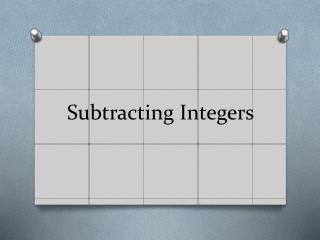DownloadDownload PresentationSubtracting Integers

# Subtracting Integers

Télécharger la présentation## Subtracting Integers

- - - - - - - - - - - - - - - - - - - - - - - - - - - E N D - - - - - - - - - - - - - - - - - - - - - - - - - - -
##### Presentation Transcript

1. Subtracting Integers

2. K-C-C • Change to an addition problem by adding the inverse (opposite). • Follow the rules for adding integers. • Ex. 14 – 32 = -18 14 – (-32) = 46 14 + (-32) = 14 + (+32) = -14 – (-32) = 18 -14 – 32 = -46 -14 + (+32) = -14 + (-32) =

3. Same sign 14 –32 = -18 56 –22 = 34 -14 –(-32) = 18 -56 – (-22)= -34 Different signs 14 – (-32) = 46 56 –(-22)= 78 -14 –32 = -46 -56 –22 = -78 AP AN

4. Same sign Subtract. -# - (-#) Change to addition problem. Keep sign of number with greatest absolute value. Hint: Even # of dashes. Different signs Add. -# - # or # - (- #) Keep sign of non-zero minuend, 1st number. Hint: Odd # of dashes.